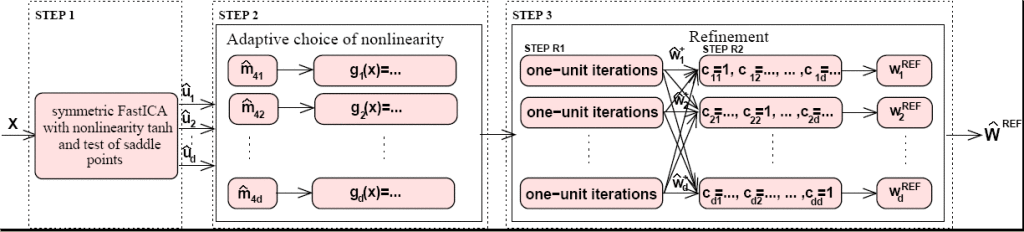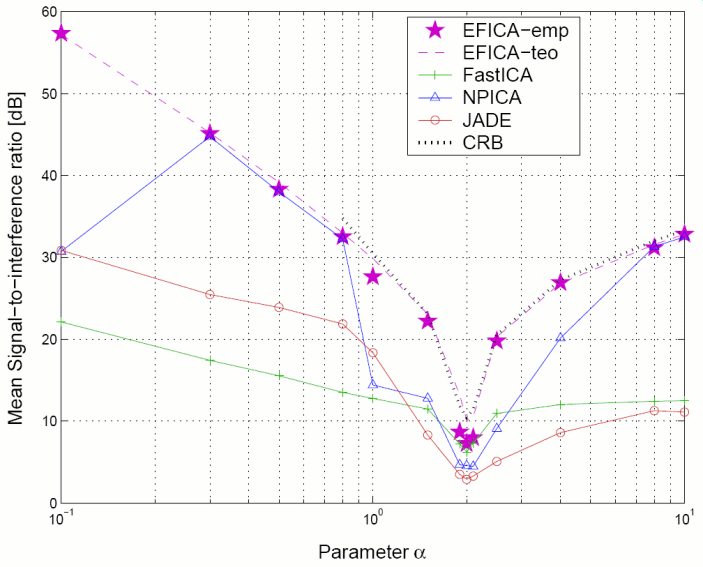# The EFICA Algorithm

Corresponding papers: journal paper, conference paper

Matlab code: here
Now version 2.12 with significantly improved speed!!!
11.6.2009 – corrected and improved Test of Saddle Points thanks to Marco Cannella from Drexel University
6.2.2009 – a bug in the refinement step was fixed

Flowchart of the algorithm:Example of utilization:

`[Wefica, ISRef]=efica(X);`
use default settings (random initialization, ‘rat2’ nonlinearity, the test of saddle points will be done)
`[Wefica, ISRef]=efica(X,eye(dim));`
identity matrix initialization, the test of saddle points will be done
`[Wefica, ISRef]=efica(X, eye(dim), false);`
identity matrix initialization, without the test of saddle points

Simulation example: 13 signals of Generalized Gaussian distribution, each with a different value of the parameter α, respectively, equal to 0.1, 0.3, 0.5, 0.8, 1, 1.5, 1.9, 2, 2.1, 2.5, 4, 8, and 10, were mixed with a random mixing matrix and demixed. The experiment was repeated 100 times with a fixed length of data N= 5000. The achieved average signal-to-interference ratios of EFICA and of other ICA methods are plotted in the following figure. Here, each value of α in the plot correspond to the result for a different original signal. CRB denotes the Cramér-Rao bound which exists for α>1.